# IDmeasurer workflow examples

#### 2019-05-09

Welcome to IDmeasurer.

## Introduction

IDmeasurer package accompanies the research article reviewing metrics that have been suggested and used to measure indivdual identity in animal signals. It allows calculation of different individual identity metrics and aims to provide common tools for scholars studying individual identity in animal signals.

The research article discussing these metrics in detail is available here.

Individual identity traits could be continuous (e.g., calling frequency, size) or discrete (e.g., presence of particular spot or pattern, presence of particular element in song). They also could be considered static (e.g., colour of an eye in humans), which remain constant or unchanged over a long time, or dynamic (e.g., voice pitch), which vary over time and depending on situation. The metrics reviewed here, in principle, could be used with any type of individual identity trait. Functions to calculate these metrics presented in this package, however, would currently be suitable for continuous and dynamic traits. The package now does not handle discrete and/or static traits, which reflects the current needs of authors’ research. It is possible that working with discrete and/or static traits would be added in future.

The package comes with the six empirical datasets which are also referred to in the research article. All of the datsets present data on individual identity in animal acoustic signals - calls and songs.

library(IDmeasurer)

The functions calculating individual identity metrics in this package, in general, use imput in form of a data frame with the first column containing individual identity codes (factor) and and with subsequent columns containing measurements of a single or several different traits (numeric). The functions to calculate identity metrics do not tranform the raw trait variables in any way so any required data transformation need to be done prior to calculating individuality metrics. The package involves six empirical datasets used in the article (ANmodulation, ANspec, CCformants, CCspec, LAhighweewoo, SSgrunts).

An example of the typical data:

summary(CCformants)
#>        id           s1f2           s1f3           s1f4           s1f5
#>  2      : 10   Min.   :1651   Min.   :2896   Min.   :3844   Min.   :5319
#>  48     : 10   1st Qu.:1996   1st Qu.:3152   1st Qu.:4649   1st Qu.:5747
#>  56     : 10   Median :2168   Median :3456   Median :4835   Median :6020
#>  59     : 10   Mean   :2194   Mean   :3614   Mean   :5014   Mean   :6187
#>  64     : 10   3rd Qu.:2362   3rd Qu.:4034   3rd Qu.:5519   3rd Qu.:6717
#>  74     : 10   Max.   :2798   Max.   :4616   Max.   :6085   Max.   :7170
#>  (Other):270
CCformants[1:20,]
#>    id s1f2 s1f3 s1f4 s1f5
#> 1   2 2668 4034 5424 6419
#> 2   2 2660 4060 5435 6416
#> 3   2 2669 4136 5532 6409
#> 4   2 2661 4103 5505 6386
#> 5   2 2657 4046 5459 6434
#> 6   2 2681 4103 5478 6434
#> 7   2 2663 4083 5491 6415
#> 8   2 2685 4089 5454 6416
#> 9   2 2677 4074 5452 6425
#> 10  2 2656 4137 5522 6416
#> 11 48 2251 4009 5503 6450
#> 12 48 2242 3961 5536 6464
#> 13 48 2247 3965 5523 6444
#> 14 48 2249 4005 5536 6427
#> 15 48 2241 3988 5549 6440
#> 16 48 2232 4027 5555 6399
#> 17 48 2268 4012 5459 6382
#> 18 48 2251 3981 5441 6405
#> 19 48 2233 4040 5507 6416
#> 20 48 2240 3987 5520 6440

Use ?CCformants etc., to get background information about datasets.

Back to Contents

## Univariate individual identity metrics

Univariate metrics quantify individual identity contained within a single trait. Function GenerateUnivariate generates univariate dataset with desired parameters.

id0 <- GenerateUnivariate(nindivs=10, nobs=10, betweenM=1000, individuality=0.01)
id3 <- GenerateUnivariate(nindivs=10, nobs=10, betweenM=1000, individuality=3)
boxplot(paramvec~id, data=id0, xlab = 'Individual no.', ylab = 'variable 1', main = 'Individuality = 0.01')
boxplot(paramvec~id, data=id3, xlab = 'Individual no.', ylab = 'variable 1', main = 'Individuality = 3')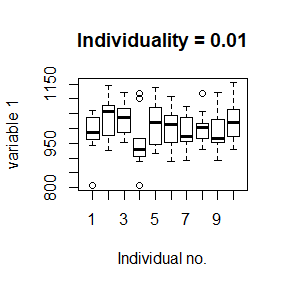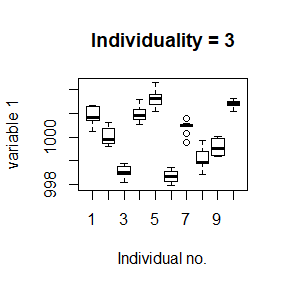In both datasets, the mean of the trait is equal to 1000. Standard deviation between individual means (SDbetween) is implicitly set to 1 in the function. Because SDbetween is fixed, individuality parameter basically changes stanadard deviation of values within individuals (SDwithin). When individuality is close to zero, within individual variation is high. Parameter individuality has to be larger than 0.

Back to Contents

### ANOVA F-value (F)

First quantification of individual identity in animal signals was done by using F-values from one-way ANOVA with individual identity as an independent variable and a trait as a dependent variable (F).

Differences between individuals are large and significant in the id3 dataset:

calcF(id0)
#>     traits    f pvals
#> 1 paramvec 1.25 0.274
calcF(id3)
#>     traits      f pvals
#> 1 paramvec 125.31     0

However, F-values reflect sample size of a dataset (number of observations for each individual) and, therefore, are not ideal for comparisons between studies with different number of observations per individual. Studies with many observations per individual will be more likely to report high individuality when using F-value as an individuality metric.

fewobs <- GenerateUnivariate(nindivs=10, nobs=10, betweenM=1000, individuality=0.5)
manyobs <- GenerateUnivariate(nindivs=10, nobs=50, betweenM=1000, individuality=0.5)
calcF(fewobs)
#>     traits   f pvals
#> 1 paramvec 3.5 0.001
calcF(manyobs)
#>     traits    f pvals
#> 1 paramvec 8.61     0

The dataset with many observations per individual manyobs has much larger F than the dataset with few observations per individual ‘fewobs’ despite the differences between individuals in both datasets are similar (Individuality = 0.5)

Because of this problem, alternative metrics were introduced.

Back to Contents

### Potential of individual coding (PIC)

Potential of individual coding (PIC) is based on the coefficent of variation which itself is sample independent. PIC is the ratio of between and within individual coefficents of variation and it is robust to number of observarions per individual (as well as to number of individuals in dataset).

calcPIC(fewobs)
#> paramvec
#> 1.119582
calcPIC(manyobs)
#> paramvec
#> 1.070051

Using PIC is fine until we want to quantify individuality in a trait which has negative and positive values because in that case coefficients of variations do not make sense.

Note that individuality in the data remains the same and we only change the mean of the trait to be 0 (and thus negative and positive values are generated around this mean). However, PIC value is strongly affected.

postrait <- GenerateUnivariate(nindivs=10, nobs=10, betweenM=1000, individuality=0.5)
posnegtrait <- GenerateUnivariate(nindivs=10, nobs=10, betweenM=0, individuality=0.5)
calcPIC(postrait)
#> paramvec
#>  1.05249
calcPIC(posnegtrait)
#> paramvec
#> 5.907797

Variants of PIC. In the literature, two variants of PIC were used depending on the way how between individual coefficient variation is calculated. Both ways can be called with functions calcPICbetweentot and calcPICbetweenmeans. The function calcPIC is equivalent to the calcPICbetweentot because values of calcPICbetweentot converge towards 1 with individuality approaching 0, and, hence, reflect the number of unique individual identity signatures in simalr way as 2^HS does.

calcPIC(id0)
#> paramvec
#> 1.019679
calcPICbetweentot(id0)
#> paramvec
#> 1.019679
calcPICbetweenmeans(id0)
#>  paramvec
#> 0.3567565

Back to Contents

### Beecher’s information statistic (HS)

Beecher’s information statistic (HS) uses ANOVA F-value but makes it sampling independent. HS is robust to number of observarions per individual as well as to number of individuals in dataset.

calcHS(fewobs)
#> HS for all vars
#>            0.16
calcHS(manyobs)
#> HS for all vars
#>             0.1

It can also handle traits with negative and positive values.

calcHS(postrait)
#> HS for all vars
#>            0.06
calcHS(posnegtrait)
#> HS for all vars
#>            0.07

This is very important advantage because it allows transforming trait variables. For example, it is possible to use Principal Component Analysis (PCA) on the raw traits and calculate HS of of the uncorrelated Principal components and sum them together to get the HS for an entire signal. Note that summing or averaging of PIC values is not a valid approach because the traits can be (and often are) correlated and, therefore, the resulting PIC values are overestimated.

Both HS and PIC are looking for the ratio of between and within individual variation and their values are closely related, but to see this, we have to convert HS into the number of potentially unique signatures. HS is expressed in bits of information and to convert it into number of signatures we have to use 2 as the base and and HS as an exponent. In that case, HS and PIC correlate almost perfectly, eventhough they are not numerically entirely equivalent.

id1 <- GenerateUnivariate(nindivs=10, nobs=10, betweenM=1000, individuality=1)
id2 <- GenerateUnivariate(nindivs=10, nobs=10, betweenM=1000, individuality=2)
id3 <- GenerateUnivariate(nindivs=10, nobs=10, betweenM=1000, individuality=3)
id4 <- GenerateUnivariate(nindivs=10, nobs=10, betweenM=1000, individuality=4)

PICs <- c(calcPIC(id1), calcPIC(id2), calcPIC(id3), calcPIC(id4))
HSs <- c(calcHS(id1), calcHS(id2), calcHS(id3), calcHS(id4))
plot((2^HSs) ~ PICs, xlim=c(0,5), ylim=c(0,5), pch=16)
abline(lm((2^HSs) ~ PICs))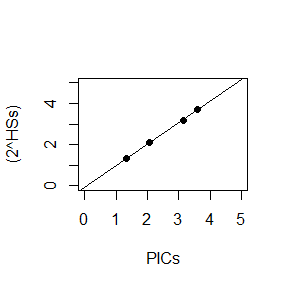Large overlap of trait values suggests low individuality

calcPIC(id4)
#> paramvec
#> 3.582682
2^calcHS(id4)
#> HS for all vars
#>        3.706352

Variants of HS. In the literature, HS has been calculated by several different approaches. These approaches are implemented in functions: calcHSntot, calcHSngroups, calcHSnpergroup, and calcHSvarcomp. calcHS is equivalent to calcHSnpergroup because it matches the assumptions stated in the original article by Beecher (1989) (see ?calcHS for the reference). Unlike the other variants, calcHSvarcomp calculates HS by ucing variance components from mixed models. This approach allows to control for possible confounding factors and variables in the model, however, we found the values to be twice as large as the values of HS calculated with calcHS.

calcHS(id3)
#> HS for significant vars         HS for all vars
#>                    1.67                    1.67
calcHSnpergroup(id3)
#> HS for significant vars         HS for all vars
#>                    1.67                    1.67
calcHSntot(id3)
#> HS for significant vars         HS for all vars
#>                    0.47                    0.47
calcHSngroups(id3)
#> HS for significant vars         HS for all vars
#>                    1.67                    1.67
calcHSvarcomp(id3)
#> HS for all vars
#>            3.34

Back to Contents

### Univariate metrics with multivariate datasets

All the three functions can be used with multivariate datasets containing the identity codes in a first column and multiple traits in subsequent columns. In that case, metrics are calculated for each trait variable. For calculation of HS for separate variables the parameter sumHS must be set to FALSE.

calcF(CCformants)
#>   traits       f pvals
#> 1   s1f2  964.26     0
#> 2   s1f3 1153.80     0
#> 3   s1f4 1089.05     0
#> 4   s1f5  818.22     0
calcPIC(CCformants)
#>     s1f2     s1f3     s1f4     s1f5
#> 10.81532 13.25913 13.89994 12.99410
calcHS(CCformants, sumHS=F)
#>   vars Pr   HS
#> 2 s1f2  0 3.30
#> 3 s1f3  0 3.43
#> 4 s1f4  0 3.39
#> 5 s1f5  0 3.19

Back to Contents

## Multivariate individual identity metrics

library(MASS)

Multivariate metrics quantify individual identity contained in multiple traits of signal. Function GenerateMultivariate generates multivariate dataset with desired parameters.

id0 <- GenerateMultivariate(nindivs=5, nobs=10, nvar=2, covar=0.9, individuality=0.01)
id5 <- GenerateMultivariate(nindivs=5, nobs=10, nvar=2, covar=0.9, individuality=5)

We generated two datasets with same properties (5 individuals, 10 observations per individual, two trait variables with covariance 0.9 between the two variables) except individuality which is low for id0 and high for id5. High individuality is clearly visible in the scatterplot of the two traits where samples belonging to the same individual are plotted with the same colour and form clearly recognizable clusters.

plot(id0[,2], id0[,3], xlab='trait 1', ylab='trait 2', pch=16, col=id0[,1])
plot(id5[,2], id5[,3], xlab='trait 1', ylab='trait 2', pch=16, col=id5[,1])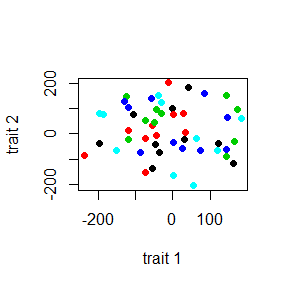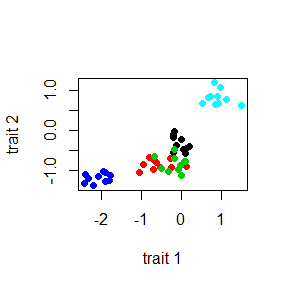Back to Contents

### Discrimination score (DS)

Most of the studies subject similar data to the discriminant function analysis and calculate discrimination score - the proportion or percentage of the samples that were classified to the correct individual.

calcDS(id0)
#>  0.16
calcDS(id5)
#>  0.86

In case of id0, individuality is very low and it is hard to discriminate individuals. Hence, the value of DS should be close to value expected by chance classification (0.2 for 5 individuals). On the other hand, discrimination is easy and DS close to maximum value of 1 in case of id5.

However, if with many individuals in the sample, it is more likely to find very similar individuals that are easy to confuse. Hence, discrimination score will be lower for large datasets. This is shown here on two datasets with the same individuality (individuality = 5) but few (nindivs = 5) and many (nindivs = 100) individuals.

id5fewinds <- GenerateMultivariate(nindivs=5, nobs=10, nvar=2, covar=0.9, individuality=5)
id5manyinds <- GenerateMultivariate(nindivs=100, nobs=10, nvar=2, covar=0.9, individuality=5)
calcDS(id5fewinds)
#>  0.94
calcDS(id5manyinds)
#>  0.276

We might try to adjust DS with regard to chance value to see how many times DS exceeds classification by chance. However, again, we do not get similar DS values for both datasets as would be expected based on the fact they are both genereated with the same individuality parameter. In this case, the bias was only reversed to favour large datasets.

calcDS(id5fewinds)/0.2 # expected classification by chance for 5 individuals: 1 / 5 = 0.2
#>  4.7
calcDS(id5manyinds)/0.01 # expected classification by chance for 5 individuals: 1 / 100 = 0.01
#>  27.6

Tranforming the raw trait values into uncorrelated principal components with Principal Component Analysis has little impact on the DS values (HS, which is discussed later, requires PCA so this is shown here for comparison):

calcDS(calcPCA(id5fewinds))/0.2 # expected classification by chance for 5 individuals: 1 / 5 = 0.2
#>  4.7
calcDS(calcPCA(id5manyinds))/0.01 # expected classification by chance for 5 individuals: 1 / 100 = 0.01
#>  27.6

DS is also biased in respect to number of individuals in the sample.

id5fewobs <- GenerateMultivariate(nindivs=10, nobs=5, nvar=2, covar=0.9, individuality=1)
id5manyobs <- GenerateMultivariate(nindivs=10, nobs=100, nvar=2, covar=0.9, individuality=1)
calcDS(id5fewobs)
#>  0.22
calcDS(id5manyobs)
#>  0.277

Like in case of F-value in univariate case, if the values are biased it makes it difficult to compare the results of different studies with various sampling of individuals and observations per individual. Therefore, alternative metrics were suggested.

Back to Contents

### Mutual information (MI)

Mutual information has been suggested as unbiased metric to complement DS. However, calcMI is subjected to the same kind of biases as DS, though these biases are reversed (like in case of DS adjusted for chance). For example, in case of number of individuals in dataset, DS will be higher for datasets with few individuals while MI will be higher for datasets with many individuals.

id5fewinds <- GenerateMultivariate(nindivs=5, nobs=10, nvar=2, covar=0.9, individuality=2)
id5manyinds <- GenerateMultivariate(nindivs=100, nobs=10, nvar=2, covar=0.9, individuality=2)
calcDS(id5fewinds)
#>  0.76
calcDS(id5manyinds)
#>  0.08
calcMI(id5fewinds)
#>  1.351647
calcMI(id5manyinds)
#>  3.412308

Back to Contents

### Beechers information statistic (HS)

HS can be used for univariate as well as for multivariate signals. It calculates and sums partial HS values of each variable. The result prints HS summed over all trait variables in dataset or over traits that are significantly different between individuals only. Since non-significant variables contribute only a little to final value of HS we suggest to report HS for all variables.

calcHS(calcPCA(id5), sumHS=T)
#> HS for significant vars         HS for all vars
#>                    3.56                    3.56

Note, that HS for multivariate signal needs to be calculated on uncorrelated variables. Here, we use the function calcPCA which uses Principal Component Analysis PCA to convert the raw trait variables into uncorrelated Principal components in the dataset. Including correlated variables overestimates the value of HS:

calcHS(id5, sumHS=T) # HS is overestimated if trait variables are correlated
#> HS for significant vars         HS for all vars
#>                    4.35                    4.35

Also note, that for good estimate of HS values the number of trait variables should not exceed the number of individuals. For discussion of this issue, see the research article introducing this package by Linhart et al. (2019).

Back to Contents

### Information capacity (HM)

HM has been suggested as variant of HS for particular type of signals where the variable traits are independent of each other. However, HS can be used for such signals as well.

id5 <- GenerateMultivariate(nindivs=10, nobs=10, nvar=2, covar=0, individuality=5)
calcHS(id5)
#> HS for significant vars         HS for all vars
#>                     4.9                     4.9

HM is an adaptation of HS and is also expressed in bits (bits of individual identity information) but the value is surprisingly different.

calcHM(id5)
#>  2.461237

It turns out that for cases in which trait variables are independent, HM calculates individual identity information per variable. In our case, we have two trait variables in signal and hance we need to multiply HM by 2 to get identity information in the entire signal. Now, the value is almost identical to HS calculated above.

calcHM(id5)*2
#>  4.922473

We can try to generate datasets with different individualities to see how the HS and HM values match each other. We will also change number of variables to 5.

id1 <- GenerateMultivariate(nindivs=10, nobs=10, nvar=5, covar=0, individuality=1)
id3 <- GenerateMultivariate(nindivs=10, nobs=10, nvar=5, covar=0, individuality=3)
id5 <- GenerateMultivariate(nindivs=10, nobs=10, nvar=5, covar=0, individuality=5)
x <- c(calcHS(id1),calcHS(id3),calcHS(id5))
y <- c(calcHM(id1)*5, calcHM(id3)*5, calcHM(id5)*5) # HM is multiplied by 5 because we have 5 trait variables.
plot(x,y)
abline(lm(y~x))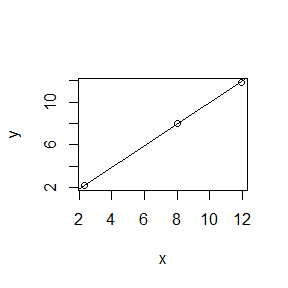Problem arises, if the trait variables are correlated.

id5covar0 <- GenerateMultivariate(nindivs=50, nobs=10, nvar=5, covar=0, individuality=5) # uncorrelated traits
id5covar1 <- GenerateMultivariate(nindivs=50, nobs=10, nvar=5, covar=1, individuality=5) # perfectly correlated traits

In such cases, HS correctly reflects lower real dimensionality of the data and hence, lower total individual identity of a signal.

calcHS(calcPCA(id5covar0))
#> HS for significant vars         HS for all vars
#>                   11.57                   11.57
calcHS(calcPCA(id5covar1))
#> HS for significant vars         HS for all vars
#>                    3.51                    3.50

Lower dimensionality is also reflected in higher overlap of values between individuals and, hence, in lower discrimination of individuals.

calcDS(calcPCA(id5covar0))
#>  0.988
calcDS(calcPCA(id5covar1))
#>  0.294

Contrary, HM remains nearly unchanged and we would need to do separate analysis of dimensionality of data to estimate identity information of the entire signal. Simple multiplying HM by number of variables would overestimate individual identity information in case that traits are correlated.

calcHM(calcPCA(id5covar0))*5 # HM is multiplied by the number of variables to get individuality of entire signal
#>  11.7644
calcHM(calcPCA(id5covar1))*5 # In this case, total individuality of entire signal is overestimated
#>  11.92642

Back to Contents

## Conversions between metrics

We believe that in future studies HS will be routinely reported as it is currently the best performing and the most universal individual identity metric available. However, in past, various metrics were used and converting between the commonly used metric could help to compare results of different studies. As we have shown earlier, PIC can be viewed as equivalent to 2^HS and, therefore, the conversion is easy in case of univariate metrics. The most frequently used metric in multivariate case was DS. To convert DS to HS and vice versa, we simulated datasets with changing parameters (number of individuals and number of observations per individual) and used loess regression model to estimate values of HS based on DS (or DS based on HS) for a particular number of individuals and number of observations per individual in the sample. Note that loess model cannot predict values outside the values used to build the model, therefore the parameters must be within:

• 5 - 40 individuals
• 5 - 20 observations per individual
• 0 - 12.9 bits for HS value
• 0 - 1 for DS value

This range of the parameters should suit to most of the published results.

HS can be estimated from DS with convertDStoHS and DS can be estimated from HS with convertHStoDS functions.

temp <- GenerateMultivariate(nindivs=20, nobs=10, nvar=2, covar=0, individuality=5)
HS <- calcHS(temp)
DS <- calcDS(temp)
HSest <- convertDStoHS(nindivs=10, nobs=10, DS=DS)
print(paste('real HS = ', HS, '; estimated HSest = ', round(HSest, 2)))
#>  "real HS =  4.71 ; estimated HSest =  4.47"
DSest <- convertHStoDS(nindivs=10, nobs=10, HS=HS)
print(paste('real DS = ', DS, '; estimated DSest = ', round(DSest, 2)))
#>  "real DS =  0.745 ; estimated DSest =  0.8"

With an argument se = TRUE, it is possible to get standard error for HS and DS estimates (note that this may take a bit longer to calculate). The estimate is then accessed with HSest$fit and standard error with HSest$se.fit calls.

Back to Contents